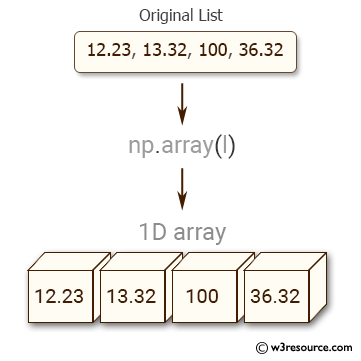﻿ NumPy: Convert a list of numeric value into a one-dimensional NumPy array - w3resource# NumPy: Convert a list of numeric value into a one-dimensional NumPy array

## NumPy: Array Object Exercise-2 with Solution

Write a NumPy program to convert a list of numeric value into a one-dimensional NumPy array.Sample Solution:-

Python Code:

``````import numpy as np
l = [12.23, 13.32, 100, 36.32]
print("Original List:",l)
a = np.array(l)
print("One-dimensional NumPy array: ",a)
```
```

Sample Output:

```Original List: [12.23, 13.32, 100, 36.32]
One-dimensional NumPy array:  [  12.23   13.32  100.     36.32]
```

numpy.array: Create an array.

Syntax: numpy.array(object, dtype=None, copy=True, order=’K’, subok=False, ndmin=0)

Parameters:

Name Description Required / Optional
object An array, any object exposing the array interface. Required
dtype : data-type, optional The desired data-type for the array. Optional
copy (bool) If true (default), then the object is copied. Optional
order : 'K', 'A', 'C', 'F' Specify the memory layout of the array. If object is not an array, the newly created array will be in C order (row major) unless ‘F’ is specified, in which case it will be in Fortran order. Optional
subok (bool) If True, then sub-classes will be passed-through, otherwise the returned array will be forced to be a base-class array. Optional
ndmin (int) Specifies the minimum number of dimensions that the resulting array should have. Optional

Python Code Editor:

Have another way to solve this solution? Contribute your code (and comments) through Disqus.

What is the difficulty level of this exercise?

Test your Python skills with w3resource's quiz

﻿

## Python: Tips of the Day

List comprehension:

```>>> m = [x ** 2 for x in range(5)]
>>> m
[0, 1, 4, 9, 16]
```